# The Lamination of Wolfram|Alpha

December 17, 2010 —Comments Off

Wolfram|Alpha already contains many extensive collections of mathematical data, including curves, surfaces, graphs, knots, and polyhedra. However, one type of object we had not systematically incorporated until recently was the class of plane geometric figures technically known as laminae: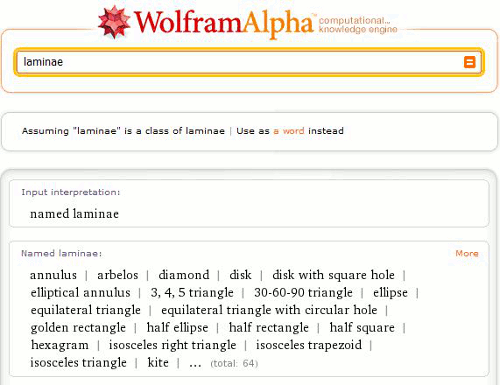Most people (including the subset of small people who play with sorting toys such as the one illustrated below) are familiar with a number of laminae. A lamina is simply a bounded (and usually connected) region of the Euclidean plane. In the most general case, it has a surface density function ?(x, y) as a function of x– and y-coordinates, but with ?(x, y) = 1 in the simplest case.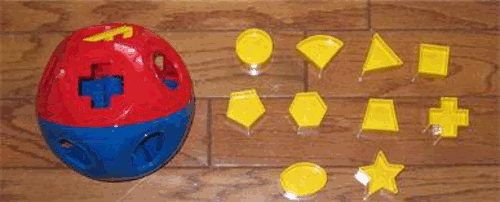Examples of laminae, some of which are illustrated above, therefore include the disk (i.e., filled circle), equilateral triangle, square, trapezoid, and 5-point star. In the interest of completeness, it might be worth mentioning that laminae are always “filled” objects, so the ambiguity about whether the terms “polygon”, “square”, etc. refer to closed sets of line segments or those segments plus their interiors does not arise for laminae.

Laminae have many interesting and useful properties. Common ones that come quickly to mind are area (say, area of an annulus):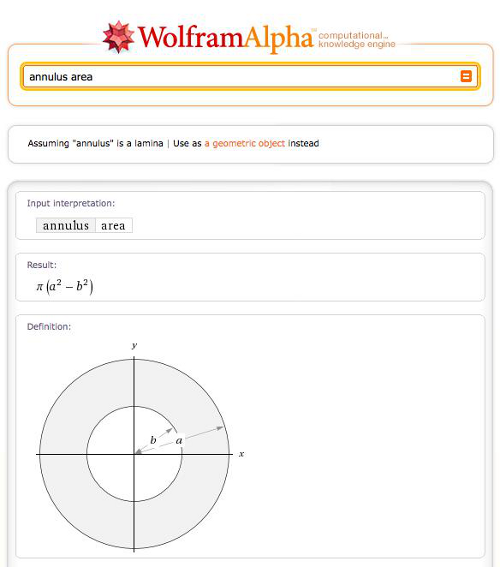And centroid (say, centroid of a parallelogram):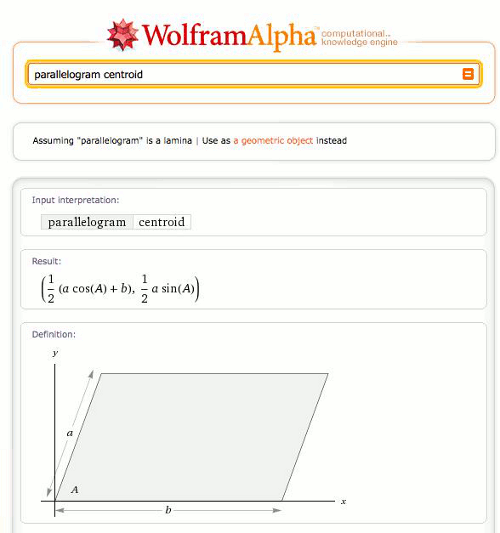As you can see, Wolfram|Alpha knows these properties. As it turns out, it also knows many other properties for both common and some not-so-common geometric figures. In addition, for each lamina, a nice schematic diagram illustrates its shape and metric parameters.

One other very useful thing Wolfram|Alpha knows about the laminae in its collection is a set of inequalities describing each. For example, octagon inequalities:

While these may seem a bit obscure, one of their great values is that Mathematica can do direct computations involving inequalities using its powerful symbolic capabilities. One important such computation is the determination of areas and centroids, both of which can be written directly as integrals over the laminar regions and hence directly computed by Mathematica. For example, the area of a regular octagon: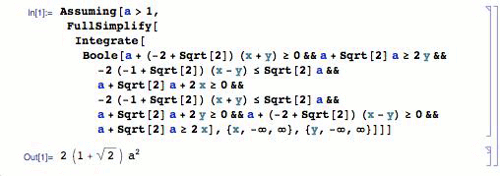Mathematica‘s ability to do computations directly on inequalities comes in especially handy when computing other, more complicated properties, such as those that occur in mechanical engineering.

In many engineering handbooks, you can find extensive listings of the mechanical and elastic properties of plates and cross-sections (both of which are—you guessed it—laminae). But Wolfram|Alpha does better than these handbooks, giving mechanical properties in exact form; for example, disk mechanical properties: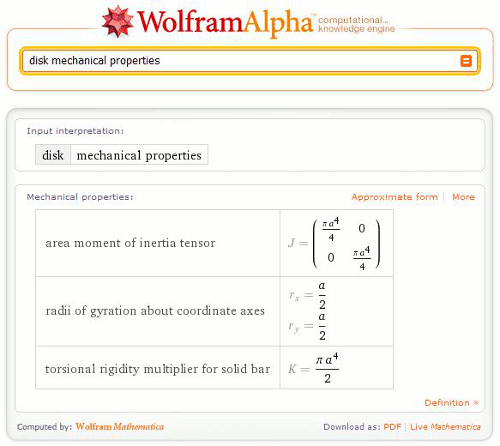Of course, after clicking the “Approximate form” button, you can also see the results in the approximate numerical form found most commonly in textbooks: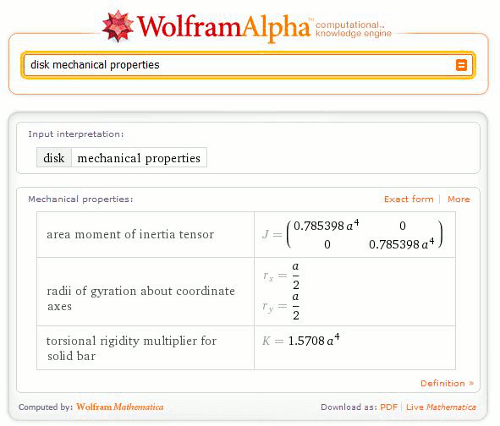Wolfram|Alpha now has a reasonable collection of beam cross sections—another type of laminae that is important in engineering applications—and their properties: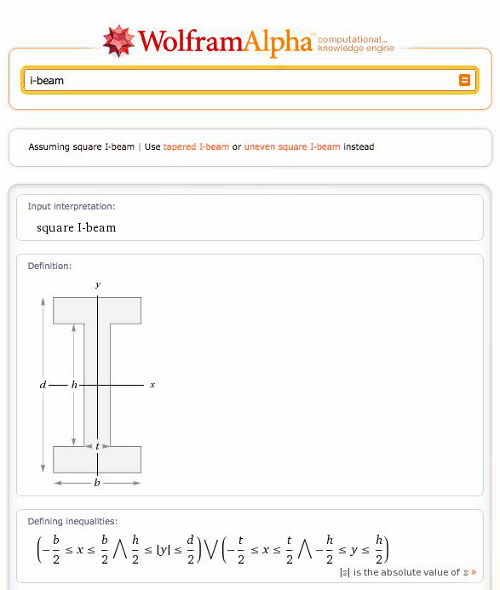There is a lot more that Wolfram|Alpha can say about laminae than I’ve been able to include in this blog, so interested mathematicians and engineers from age 1 to 100 should feel free to explore further the computable properties of laminae in Wolfram|Alpha.